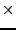Next: Procedure for following branches Up: Implementation Previous: Implementation   Contents

Certified Newton

The procedure that allow to determine the next point on a branch is implemented as:

int Certified_Newton(int Nb_Var,int Dimension_Eq,int Nb_Branch,int Branch,
INTERVAL_VECTOR (* Func)(int,int,INTERVAL_VECTOR &),
INTERVAL_MATRIX (* Hessian)(int, int, INTERVAL_VECTOR &),
double Accuracy_Var,double Accuracy,
double *param,double delta_param,double min_delta_param,
int sens,MATRIX &Solution)
This procedure determine if it possible to find a new point on the branch numbered Branch among the Nb_Branch that have been found. In Solution we have all the solutions that have been found for the branches when the parameter value was param. The change in the parameter value will be at most sensdelta_param and on exit of the procedure param will be set to the new value. sens indicates the direction of change in the parameter value: +1 for an increase, -1 for a decrease.

This procedure returns:

• -1, -4: even with a change of the parameter value of min_delta_param we cannot either distinguish two solutions or Kantorovitch has failed to give a positive answer. But this does not mean in general that a singularity occurs.
• -2: for systems having more equations than unknowns the solution that has been obtained with Newton for the square system of equations failed to cancel the remaining equations
• -3: Newton has not converged (should not occur)
• -10: singular point
• 1, 2: procedure has succeededNext: Procedure for following branches Up: Implementation Previous: Implementation   Contents
Jean-Pierre Merlet 2012-12-20